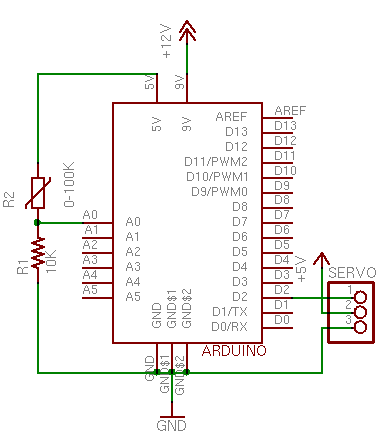# Expand Your Creativity Horizon Starting with the Servo Motor Today

Servomotor is one of the most common electronic components, and you can control the servomotor position by only using the value returned from analog sensor. Servos also the easiest way to get you started with a microcontroller related with motion.

Those components you needed are:

• 22-AWG hookup wire
• Arduino microcontroller board
• 10K ohm resistors
• Flex sensors, and of course
• RC ServomotorOk, let’s start the project. Firstly, you need to connect power and ground on the breadboard to power and ground from the microcontroller. Use the 5V and any of the ground connections on the Arduino module (Please refer the above figure).Afterward, pick any analog input and connect it to Analog pin 0.  Also, try to connect an RC servomotor to the digital pin 2 (Remember, the yellow wire of the servo goes to the pin, while the red and black wires must go to +5V and ground).

Here is the programming code for this project:

`/*`
` Servo control from an analog input`
`The minimum (minPulse) and maxiumum (maxPuluse) values`
`will be different depending on your specific servo motor.`
`Ideally, it should be between 1 and 2 milliseconds, but in practice,`
`0.5 - 2.5 milliseconds works well for me.`
`Try different values to see what numbers are best for you.`
`This program uses the millis() function to keep track of when the servo was`
`last pulsed.  millis() produces an overflow error (i.e. generates a number`
`that's too big to fit in a long variable) after about 5 days. if you're`
`making a program that has to run for more than 5 days, you may need to`
`account for this.`
`by Tom Igoe`
`additions by Carlyn Maw & Rob Faludi`
`Created 28 Jan. 2006`
`Updated 10 Jun. 2008`
`*/`
`int servoPin = 2;     // Control pin for servo motor`
`int minPulse = 500;   // Minimum servo position`
`int maxPulse = 2500;  // Maximum servo position`
`int pulse = 0;        // Amount to pulse the servo`
`long lastPulse = 0;    // the time in milliseconds of the last pulse`
`int refreshTime = 20; // the time needed in between pulses`
`int analogValue = 0;  // the value returned from the analog sensor`
`int analogPin = 0;    // the analog pin that the sensor's on`
` void setup() {`
` pinMode(servoPin, OUTPUT);  // Set servo pin as an output pin`
` pulse = minPulse;           // Set the motor position value to the minimum`
` Serial.begin(9600);`
`}`
` void loop() {`
` analogValue = analogRead(analogPin);      // read the analog input`
` pulse = map(analogValue,0,1023,minPulse,maxPulse);    // convert the analog value`
`                                                       // to a range between minPulse`
`                                                       // and maxPulse.`
`  // pulse the servo again if rhe refresh time (20 ms) have passed:`
` if (millis() - lastPulse >= refreshTime) {`
`   digitalWrite(servoPin, HIGH);   // Turn the motor on`
`   delayMicroseconds(pulse);       // Length of the pulse sets the motor position`
`   digitalWrite(servoPin, LOW);    // Turn the motor off`
`   lastPulse = millis();           // save the time of the last pulse`
` }`
`}`

Since the code was well written with a potentiometer, it automatically assumed you’re going to get values from 0 to 1023 from the sensor.

Lastly, do test the approximation at the extremes of the both sensor and the output, for maker sure it does its job.